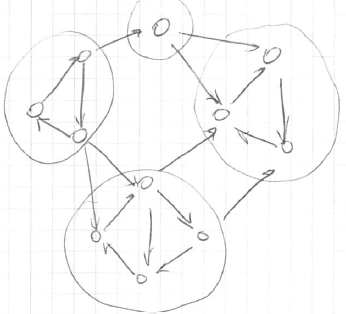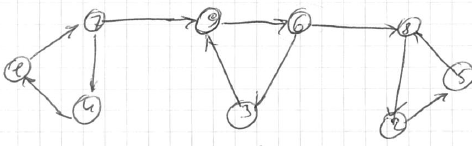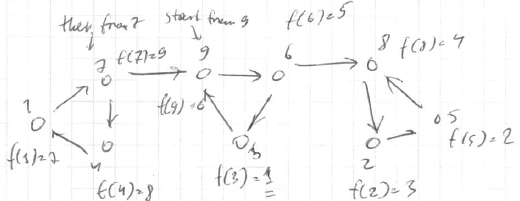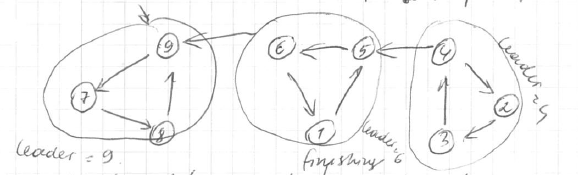# ML Wiki

## Computing Strong Components

strongly connected:

• you can get to any point to any point within component
• exampleidea:

• use DFS and mark "leaders"
• leaders - points where DFS starts
• nodes with the same leader are SCCs

where to start?

• it depends on the starting point
• with good starting point we may discover a SCC
• with bad - the whole graph

## Kosaraju's Two-Pass algorithm

This is a randomized algorithm for finding strongly connected components, consists of 2 DFS passes of the graph.

### First pass

• compute the "magical" ordering: the finishing times
• reverse the graph and run DFS

Example

• graph (already reversed)• t = [7, 3, 1, 8, 2, 5, 9, 4, 6]
• order### Second pass

• now we replace ordinal node names with finishing times
• and it's run on the original graph (not the reversed)

Example

• second pass### Algorithm

It consists of 3 routines: Kosaraju's, DFS-loop and DFS

Kosaraju's:

• let $G_{\text{rev}}$ be $G$ with all arcs reversed
• run DFS-loop on $G_{\text{rev}}$ to compute magic ordering of nodes
• let $f(v)$ = "finishing time"
• run DFS-loop on $G$ and process nodes in decreasing order of their finishing time

DFS-loop(graph $G$):

• global $t$ = 0: number of nodes processed so far
• global $s$ = NULL: most recent node from which DFS initiated
• for $i$ = $n$ downto $1$
• if $i$ not explored
• $s$ = $i$
• DFS($G$, $i$)

DFS(graph $G$, node $i$):

• mark $i$ explored
• leader($i$) = node $s$ (where DFS started)
• for each $(i, j) \in G$
• if $j$ not explored
• DFS($G$, $j$)
• $t$ = $t$ + $1$: finishing time
• set $f(i)$ = $t$

Running time: 2DFS = $O(n + m)$

### Correctness

idea of the correctness:

• maximal finishing time is a sink
• if we replace each SCC with just a node
• the sink won't have outgoing edges
• first pass finds the sink SCC
• second pass "peels off" SCCs one-by-one

### Implementation

public class StronglyConnectedComponents {
private Graph graph;

private int counter = 0;

private boolean visited[];
private int finishingTime[];
private int finishingTimeReversed[];

public void run() {
dfs1Loop();
dfs2Loop();
}

public void dfs1Loop() {
visited = new boolean[graph.getN()];
finishingTime = new int[graph.getN()];
Arrays.fill(finishingTime, -1);
finishingTimeReversed = new int[graph.getN()];
Arrays.fill(finishingTimeReversed, -1);

for (int i = graph.getN() - 1; i >= 0; i--) {
if (!visited[i]) {
dfs1(i);
}
}
}

private void dfs1(int u) {
visited[u] = true;

for (int v : graph.reverse(u)) {
if (!visited[v]) {
dfs1(v);
}
}

finishingTime[u] = counter;
finishingTimeReversed[counter] = u;
counter++;
}

public void dfs2Loop() {
visited = new boolean[graph.getN()];

for (int i = graph.getN() - 1; i >= 0; i--) {
int ft = finishingTimeReversed[i];
if (!visited[ft]) {
dfs2(ft);
}
}
}

private void dfs2(int u) {
visited[u] = true;

for (int v : graph.adjacent(u)) {
if (!visited[v]) {
dfs2(v);
}
}
}
}